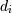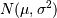# numpy.random.RandomState.randn¶

RandomState.randn(d0, d1, ..., dn)

Return a sample (or samples) from the “standard normal” distribution.

If positive, int_like or int-convertible arguments are provided, randn generates an array of shape (d0, d1, ..., dn), filled with random floats sampled from a univariate “normal” (Gaussian) distribution of mean 0 and variance 1 (if any of theare floats, they are first converted to integers by truncation). A single float randomly sampled from the distribution is returned if no argument is provided.

This is a convenience function. If you want an interface that takes a tuple as the first argument, use numpy.random.standard_normal instead.

Parameters: d0, d1, ..., dn : int, optional The dimensions of the returned array, should be all positive. If no argument is given a single Python float is returned. Z : ndarray or float A (d0, d1, ..., dn)-shaped array of floating-point samples from the standard normal distribution, or a single such float if no parameters were supplied.

random.standard_normal
Similar, but takes a tuple as its argument.

Notes

For random samples from, use:

sigma * np.random.randn(...) + mu

Examples

>>> np.random.randn()
2.1923875335537315 #random


Two-by-four array of samples from N(3, 6.25):

>>> 2.5 * np.random.randn(2, 4) + 3
array([[-4.49401501,  4.00950034, -1.81814867,  7.29718677],  #random
[ 0.39924804,  4.68456316,  4.99394529,  4.84057254]]) #random


#### Previous topic

numpy.random.RandomState.randint

#### Next topic

numpy.random.RandomState.random_integers